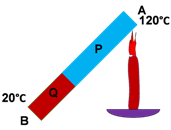# Fisika Study Center

## 9 Common Problems of Heat

physics.fisikastudycenter.com - 9 common problems of heat, discussed at grade 10 of high school physics. Included heat,coefficients of thermal expansion, length expansion, area expansion and volume expansion, heat transfer.Problem 1
A metal of 2500 g of mass takes 12 kJ of heat to raise its temperature. If the specific heat of this metal is 0,2 kalori/groC and its initial temperature is 30oC, find the final temperature of this metal!

Answer
Given :
Q = 12 kilojoule = 12000 joule
m = 2500 gram = 2,5 kg
T1 = 30oC
c = 0,2 cal/groC = 0,2 x 4200 joule/kg oC = 840 joule/kg oC
T2 =...?

Q = mcΔT
12000 = (2,5)(840)ΔT
ΔT = 12000/2100 = 5,71 oC

T2 = T1 + ΔT = 30 + 5,71 = 35,71 oC

Problem 2
500 g of ice at −12oC is heated to −2oC. If the specific heat of ice is 0,5 cal/goC, find the amount of heat required for this process, express your answer in joule!

Answer
Data :
m = 500 gram
T1 = −12oC
T2 = −2oC
ΔT = T2 − T1 = −2o − (−12 ) = 10oC
c = 0,5 calorie/groC
Q = ....?

Q = mcΔT
Q = (500)(0,5)(10) = 2500 calorie

1 calorie = 4,2 joule
Q = 2500 x 4,2 = 10500 joule

Problem 3
How much heat is required to change 500 g of ice at 0oC to liquid water at 0oC if the specific heat of ice is 0,5 cal/goC and the ice latent heat of fusion is 80 cal/g, show the answer in kilocalorie

Answer
Values given:
m = 500 gram
L = 80 calori/gr
Q = ....?

Q = mL
Q = (500)(80) = 40000 calori = 40 kcal

Soal No. 4
How much heat is required to change 500 g of ice at 0oC to liquid water of 5oC, if the specific heat of ice is 0,5 cal/goC, the specifc heat of water is 1 cal/goC and the ice heat of fusion is 80 cal/g!

Answer
The given values are:
m = 500 gram
cwater = 1 calori/groC
Lice = 80 calori/gr
Final temperature → 5oC
Q = .....?

There are two steps in here
→ melting the ice , Q1 needed
Q1 = mLice = (500)(80) = 40000 calori

→ raising water temperature from 0oC to 5oC, Q2 needed
Q2 = mcwaterrΔTwater = (500) (1)(5) = 2500 calori

Kalor total yang diperlukan:
Q = Q1 + Q2 = 40000 + 2500 = 42500 calori

Problem 5
Determine the the heat needed to change 500 g of ice at −10oC to 5oC of liquid water!

Answer
Data yang diperlukan:
m = 500 gram
cice = 0,5 calori/groC
cwater = 1 cal/groC
Lice = 80 cal/gr
final temperature → 5oC
Q = .....?

There are three steps in here:
→ raising ice temperature fromi −10oC 0oC, Q1 needed
Q1 = mcesΔTes = (500)(0,5)(10) = 2500 calorie

→ melting ice , Q2 needed
Q2 = mLice = (500)(80) = 40000 calorie

→ raising water temperature from 0oC to 5oC, Q3 needed
Q3 = mcwaterΔTwater = (500)(1)(5) = 2500 kalori

So then
Q = Q1 +Q2 + Q3 = 2500 + 40000 + 2500 = 45000 calorie

Problem 6
What is the final temperature of a mixture contents 200 g of water at 80oC and 300 g of water at 20oC?

Answer
Given values
m1 = 200 gram
m2 = 300 gram
ΔT1 = 80 − t
ΔT2 = t − 20
final temperature = t = ......?

Qreleased = Qtaken
m1c1ΔT1 = m2c2ΔT2
(200)(1) (80 − t) = (300)(1)(t − 20)
(2)(1)(80 − t) = (3)(1)(t − 20)
160 − 2t = 3t − 60
5t = 220
t = 44oC

Problem 7
You have a cup contents 200 g of water at 50°C. Your sister puts 100 g of ice at 0°C into the cup. Determine the final temperature of the water, assume that no heat is lost to the surroundings and no heat is taken by your cup!< br />

Use the specific heat heat of water 1 cal/gr°C, for ice 0,5 cal/gr°C, ice heat of fusion is 80 cal/gr.

Answer
The heat released by water (Q1) is the same in amount with the heat taken by ice to melt (Q2) and to raise temperature (Q3)Problem 8
Given two bars of metals made from the same materials configured as below figure.The lenght of P is doubles of the length of Q and the thermal conductivity of P is a half of Q. Determine the temperature of the juntion of the two bars!

Answer
Heat flow through two bars of same composition.Soal No. 9
A steel tank with its coefficient of linear expansion 12 x 10-6/°C, and its volume 0,05 m3 is full of gasoline. The tank then is heated from 20°C. Find the volume of gasoline overflows from the tank when the temperature raise to 50°C, use the coefficient of volume expansion of gasoline 950 x 10-6/°C !

Answer

The volume of gasoline that overflowsprepared by:
fisikastudycenter.com

Joomla Templates: by JoomlaShack
Template Upgrade by Joomla Visually